Download Presentation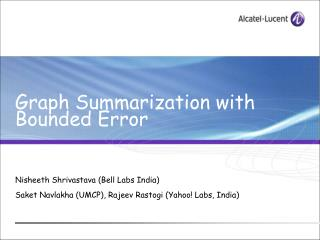Graph Summarization with Bounded Error

# Graph Summarization with Bounded Error - PowerPoint PPT Presentation

Graph Summarization with Bounded Error. Nisheeth Shrivastava (Bell Labs India) Saket Navlakha (UMCP), Rajeev Rastogi (Yahoo! Labs, India). E. F. yahoo.com. cnn.com. 20.20.2.2. D. B. A. C. 10.1.1.1. G. Netflow. jokes.com. Social Networks. Large Graphs.I am the owner, or an agent authorized to act on behalf of the owner, of the copyrighted work described.
Download Presentation## Graph Summarization with Bounded Error

An Image/Link below is provided (as is) to download presentation

Download Policy: Content on the Website is provided to you AS IS for your information and personal use and may not be sold / licensed / shared on other websites without getting consent from its author.While downloading, if for some reason you are not able to download a presentation, the publisher may have deleted the file from their server.

- - - - - - - - - - - - - - - - - - - - - - - - - - E N D - - - - - - - - - - - - - - - - - - - - - - - - - -
Presentation Transcript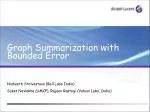### Graph Summarization with Bounded Error

Nisheeth Shrivastava (Bell Labs India)

Saket Navlakha (UMCP), Rajeev Rastogi (Yahoo! Labs, India)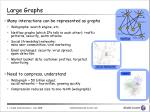E

F

yahoo.com

cnn.com

20.20.2.2

D

B

A

C

10.1.1.1

G

Netflow

jokes.com

Social Networks

Large Graphs
• Many interactions can be represented as graphs
• Webgraphs: search engine, etc.
• Netflow graphs (which IPs talk to each other): traffic patterns, security, worm attacks
• Social (friendship) networks: mine user communities, viral marketing
• Email exchanges: security. virus spread, spam detection
• Market basket data: customer profiles, targeted advertizing
• Need to compress, understand
• Webgraph ~ 50 billion edges; social networks ~ few million, growing quickly
• Compression reduces size to one-tenth (webgraphs)

email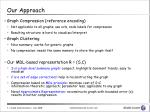Our Approach
• Graph Compression (reference encoding)
• Not applicable to all graphs: use urls, node labels for compression
• Resulting structure is hard to visualize/interpret
• Graph Clustering
• Nice summary, works for generic graphs
• No compression: needs the same memory to store the graph itself
• Our MDL-based representation R = (S,C)
• S is a high-level summary graph: compact, highlights dominant trends, easy to visualize
• C is a set of edge corrections: help in reconstructing the graph
• Compression based on MDL principle:minimize cost of S+C information-theoretic approach; parameter less; applicable to any graph
• Novel Approximate Representation: reconstructs graph with bounded error (є); results in better compression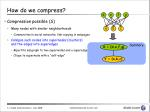How do we compress?

d

e

f

g

• Compression possible (S)
• Many nodes with similar neighborhoods
• Communities in social networks; link-copying in webpages
• Collapse such nodes into supernodes (clusters) and the edges into superedges
• Bipartite subgraph to two supernodes and a superedge
• Clique to supernode with a “self-edge”

a

b

c

Summary

X = {d,e,f,g}

Y = {a,b,c}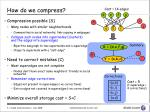i

h

j

i

h

i

How do we compress?

Cost = 14 edges

d

e

f

g

• Compression possible (S)
• Many nodes with similar neighborhoods
• Communities in social networks; link-copying in webpages
• Collapse such nodes into supernodes (clusters) and the edges into superedges
• Bipartite subgraph to two supernodes and a superedge
• Clique to supernode with a “self-edge”
• Need to correct mistakes (C)
• Most superedges are not complete
• Nodes don’t have exact same neighbors: friends in social networks
• Remember edge-corrections
• Edges not present in superedges (-ve corrections)
• Extra edges not counted in superedges (+ve corrections)
• Minimize overall storage cost = S+C

a

b

c

Summary

X = {d,e,f,g}

Y = {a,b,c}

Corrections

Cost = 5(1 superedge + 4 corrections)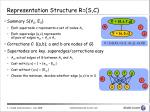Representation Structure R=(S,C)

X = {d,e,f,g}

• Summary S(VS, ES)
• Each supernode v represents a set of nodes Av
• Each superedge (u,v) represents all pair of edges πuv = Au x Av
• Corrections C: {(a,b); a and b are nodes of G}
• Supernodes are key, superedges/corrections easy
• Auv actual edges of G between Au and Av
• Cost with (u,v) = 1 + |πuv – Euv|
• Cost without (u,v) = |Euv|
• Choose the minimum, decides whether edge (u,v) is in S

h

i

Y = {a,b,c}

j

C = {+(a,h), +(c,i), +(c,j), -(a,d)}

d

e

f

g

h

i

j

a

b

c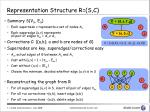Representation Structure R=(S,C)

X = {d,e,f,g}

• Summary S(VS, ES)
• Each supernode v represents a set of nodes Av
• Each superedge (u,v) represents all pair of edges πuv = Au x Av
• Corrections C: {(a,b); a and b are nodes of G}
• Supernodes are key, superedges/corrections easy
• Auv actual edges of G between Au and Av
• Cost with (u,v) = 1 + |πuv – Euv|
• Cost without (u,v) = |Euv|
• Choose the minimum, decides whether edge (u,v) is in S
• Reconstructing the graph from R
• For all superedges (u,v) in S, insert all pair of edges πuv
• For all +ve corrections +(a,b), insert edge (a,b)
• For all -ve corrections -(a,b), delete edge (a,b)

h

i

Y = {a,b,c}

j

C = {+(a,h), +(c,i), +(c,j), -(a,d)}

d

e

f

g

h

i

j

a

b

c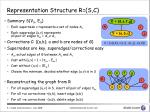Representation Structure R=(S,C)

X = {d,e,f,g}

• Summary S(VS, ES)
• Each supernode v represents a set of nodes Av
• Each superedge (u,v) represents all pair of edges πuv = Au x Av
• Corrections C: {(a,b); a and b are nodes of G}
• Supernodes are key, superedges/corrections easy
• Auv actual edges of G between Au and Av
• Cost with (u,v) = 1 + |πuv – Euv|
• Cost without (u,v) = |Euv|
• Choose the minimum, decides whether edge (u,v) is in S
• Reconstructing the graph from R
• For all superedges (u,v) in S, insert all pair of edges πuv
• For all +ve corrections +(a,b), insert edge (a,b)
• For all -ve corrections -(a,b), delete edge (a,b)

h

i

Y = {a,b,c}

j

C = {+(a,h), +(c,i), +(c,j), -(a,d)}

d

e

f

g

h

i

j

a

b

c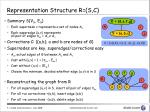Representation Structure R=(S,C)

X = {d,e,f,g}

• Summary S(VS, ES)
• Each supernode v represents a set of nodes Av
• Each superedge (u,v) represents all pair of edges πuv = Au x Av
• Corrections C: {(a,b); a and b are nodes of G}
• Supernodes are key, superedges/corrections easy
• Auv actual edges of G between Au and Av
• Cost with (u,v) = 1 + |πuv – Euv|
• Cost without (u,v) = |Euv|
• Choose the minimum, decides whether edge (u,v) is in S
• Reconstructing the graph from R
• For all superedges (u,v) in S, insert all pair of edges πuv
• For all +ve corrections +(a,b), insert edge (a,b)
• For all -ve corrections -(a,b), delete edge (a,b)

h

i

Y = {a,b,c}

j

C = {+(a,h), +(c,i), +(c,j), -(a,d)}

d

e

f

g

h

i

j

a

b

c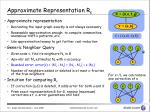Approximate Representation Rє

X = {d,e,f,g}

• Approximate representation
• Recreating the input graph exactly is not always necessary
• Reasonable approximation enough: to compute communities, anomalous traffic patterns, etc.
• Use approximation leeway to get further cost reduction
• Generic Neighbor Query
• Given node v, find its neighbors Nv in G
• Apx-nbr set N’v estimates Nv with є-accuracy
• Bounded error: error(v) = |N’v - Nv| + |Nv - N’v| < є |Nv|
• Number of neighbors added or deleted is at most є-fraction of the true neighbors
• Intuition for computing Rє
• If correction (a,d) is deleted, it adds error for both a and d
• From exact representation R for G, remove (maximum) corrections s.t. є-error guarantees still hold

Y = {a,b}

C = {-(a,d), -(a,f)}

d

e

f

g

G

a

b

For є=.5, we can remove one correction of a

d

e

f

g

a

bComparison with existing techniques
• Webgraph compression [Adler-DCC-01]
• Use nodes sorted by urls: not applicable to other graphs
• More focus on bitwise compression: represent sequence of neighbors (ids) using smallest bits
• Clique stripping [Feder-pods-99]
• Collapses edges of complete bi-partite subgraph into single cluster
• Only compresses very large, complete bi-cliques
• Representing webgraphs [Raghavan-icde-03]
• Represent webgraphs as SNodes, Sedges
• Use urls of nodes for compression (not applicable for other graphs)
• No concept of approximate representation

d

e

f

g

a

b

c

d

e

f

g

a

b

cOutline
• Compressed graph
• MDL representation R=(S,C); є-representation
• Computing R
• GREEDY, RANDOMIZED
• Computing Rє
• APX-MDL, APX-GREEDY
• Experimental results
• Conclusions and future workGREEDY
• Cost of merging supernodes u and v into single supernode w
• Recall: cost of a superedge (u,x): c(u,x) = min{|πvx – Avx|+1, |Avx|}
• cu = sum of costs of all its edges = Σx c(u,x)
• s(u,v) = (cu + cv – cw)/(cu + cv)
• Main idea: recursive bottom-up merging of supernodes
• If s(u,v) > 0, merging u and v reduces the cost of reduction
• Normalize the cost: remove bias towards high degree nodes
• Making supernodes is the key: superedges and corrections can be computed later

u

v

w

cu = 5; cv =4

cw = 6 (3 edges, 3 corrections)

s(u,v) = 3/9GREEDY

Cost reduction: 11 to 6

bc

• Recall: s(u,v) = (cu + cv – cw)/(cu + cv)
• GREEDY algorithm
• At every step, pick the pair with max s(.) value, merge them
• If no pair has positive s(.) value, stop

a

d

ef

gh

C = {+(h,d),+(a,e)}

b

bc

bc

c

a

a

a

d

d

d

e

e

e

h

h

f

gh

f

f

g

g

C = {+(h,d)}

s(b,c)=.5[ cb = 2; cc=2; cbc=2 ]

s(g,h)=3/7[ cg = 3; ch=4; cgh=4 ]

s(e,f)=1/3[ ce = 2; cf=1; cef=2 ]RANDOMIZED
• GREEDY is slow
• Need to find the pair with (globally) max s(.) value
• Need to process all pair of nodes at a distance of 2-hops
• Every merge changes costs of all pairs containing Nw
• Main idea: light weight randomized procedure
• Instead of choosing the globally best pair,
• Choose (randomly) a node u
• Merge the best pair containing uRANDOMIZED

b

c

a

d

• Randomized algorithm
• Unfinished set U=VG
• At every step, randomly pick a node u from U
• Find the node v with max s(u,v) value
• If s(u,v) > 0, then merge u and v into w, put w in U
• Else remove u from U
• Repeat till U is not empty

e

h

f

g

Picked e; s(e,f)=3/5[ ce = 3; cf=2; cef=3 ]

b

c

a

d

h

ef

g

C = {+(a,e)}Outline
• Compressed graph
• MDL representation R=(S,C); є-representation
• Computing R
• GREEDY, RANDOMIZED
• Computing Rє
• APX-MDL, APX-GREEDY
• Experimental results
• Conclusions and future workComputing approx representation

S

• Reducing size of corrections
• Correction graph H: For every (+ve or –ve) correction (a,b) in C, add edge (a,b) to H
• Removing (a,b) reduces size of C, but adds error of 1 to a and b
• Recall bounded error: error(v) = |N’v - Nv| + |Nv - N’v| < є |Nv|
• Implies in H, we can remove up to bv = є |Nv| edges incident on v
• Maximum cost reduction: remove subset M of EH of max size s. t. M has at most bv edges incident on v
• Same as the b-matching problem
• Find the matching M\subset EG s.t. at most bv edges incident on v are in M
• For all bv = 1, traditional matching problem
• Solvable in time O(mn2) [Gabow-STOC-83] (for graph with n nodes and m edges)

CComputing approx representation

S

• Reducing size of summary
• Removing superedge (a,b) implies bulk removal of all pair edges πuv
• But, each node in Au and Av has different b value
• Does not map to a clean matching-type problem
• A greedy approach
• Pick superedges by increasing |πuv| value
• Delete (u,v) if that doesn’t violate є-bound for nodes in AuUAv
• If there is correction (a,b) for πuv in C, we cannot remove (u,v); since removing (u,v) violates error bound for a or bAPXMDL

S

C

• Compute the R(S,C) for G
• Find Cє
• Compute H, with VH=C
• Find maximum b-matching M for H; Cє=C-M
• Find Sє
• Pick superedges (u,v) in S having no correction in Cєin increasing |πuv| value
• Remove (u,v) if that doesn’t violate є-bound for any node in Au U Av
• Axp-representation Rє=(Cє, Sє)Outline
• Compressed graph
• MDL representation R=(S,C); є-representation
• Computing R
• GREEDY, RANDOMIZED
• Computing Rє
• APX-MDL, APX-GREEDY
• Experimental results
• Conclusions and future workExperimental set-up
• Algorithms to compare
• Our techniques GREEDY, RANDOMIZED, APXMDL
• REF: reference encoding used for web-graph compression (we disabled bit-level encoding techniques)
• GRAC: graph clustering algorithm (make supernodes for clusters returned)
• Datasets
• CNR: web-graph dataset
• Routeview: autonomous systems topology of the internet
• Wordnet: English words, edges between related words (synonym, similar, etc.)
• Facebook: social networkingCost Reduction (CNR dataset)

Reduces the cost down to 40%

Cost of GREEDY 20% lower than RANDOMIZED

RANDOMIZED is 60% faster than GREEDYComparison with other schemes

Our techniques give much better compressionCost Breakup (CNR dataset)

80% cost of representation is due to correctionsApx-Representation

Cost reduces linearly as єis increased;

With є=.1, 10% cost reduction over RConclusions
• MDL-based representation R(S,C) for graphs
• Compact summary S: highlights trends
• Corrections C: reconstructs graph together with S
• Extend to approximate representation with bounded error
• Our techniques, GREEDY, RANDOMIZED give up to 40% cost reduction
• Future directions
• Hardness of finding minimum-cost representation
• Running graph algorithms (approximately) directly on the compressed structure: apx-shortest path with bounded error on S?
• Extend to labeled/weighted edges